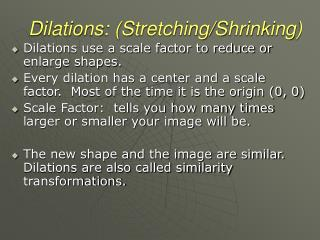Download PresentationDilations: (Stretching/Shrinking)

Dilations: (Stretching/Shrinking) - PowerPoint PPT Presentation

Dilations: (Stretching/Shrinking). Dilations use a scale factor to reduce or enlarge shapes. Every dilation has a center and a scale factor. Most of the time it is the origin (0, 0) Scale Factor: tells you how many times larger or smaller your image will be.I am the owner, or an agent authorized to act on behalf of the owner, of the copyrighted work described.
Download PresentationDilations: (Stretching/Shrinking)

Download Policy: Content on the Website is provided to you AS IS for your information and personal use and may not be sold / licensed / shared on other websites without getting consent from its author.While downloading, if for some reason you are not able to download a presentation, the publisher may have deleted the file from their server.

- - - - - - - - - - - - - - - - - - - - - - - - - - E N D - - - - - - - - - - - - - - - - - - - - - - - - - -
Presentation Transcript
1. Dilations: (Stretching/Shrinking) • Dilations use a scale factor to reduce or enlarge shapes. • Every dilation has a center and a scale factor. Most of the time it is the origin (0, 0) • Scale Factor: tells you how many times larger or smaller your image will be. • The new shape and the image are similar. Dilations are also called similarity transformations.

2. How do we locate dilation images? • A dilation is a transformation whose preimage and image are similar. • Every dilation has a center and a scale factor n, n >0. The scale factor describes the size change from the original figure to the image.

3. Example 1: • Quadrilateral ABCD has vertices A(-2, -1), B(-2, 1), C(2, 1) and D(1, -1). • Find the coordinates of the image for the dilation with a scale factor of 2 and center of dilation at the origin. C’ B’ B C A D A’ D’

4. F(-3, -3), O(3, 3), R(0, -3) Scale factor 1/3 Example 2: O O’ F’ R’ F R

5. T(-1, 0), H(1, 0), I(2, -2), S(-2, -2) Scale factor 4 Example 3: T H H’ T’ S I I’ S’

6. The dilation is an enlargement if the scale factor is > 1. The dilation is a reduction if the scale factor is between 0 and 1.

7. Finding a Scale Factor • The blue triangle is a dilation image of the red triangle. Describe the dilation. • The center is X. The image is larger than the preimage, so the dilation is an enlargement.

8. Finding a Scale Factor • The blue quadrilateral is a dilation image of the red quadrilateral. Describe the dilation.

9. Graphing Dilation Images • ∆PZG has vertices P(2,0), Z(-1, ½), and G (1, -2). What are the coordinates of the image of P for a dilation with center (0,0) and scale factor 3? a) (5, 3) b) (6,0) c) (2/3, 0) d) (3, -6)

10. Solution: The scale factor is 3, so use the rule: (x, y)(3x, 3y). P(2,0) P’(3•2, 3•0) or P’(6, 0). The correct answer is B. What are the coordinates for G’ and Z’? Graphing Dilation Images